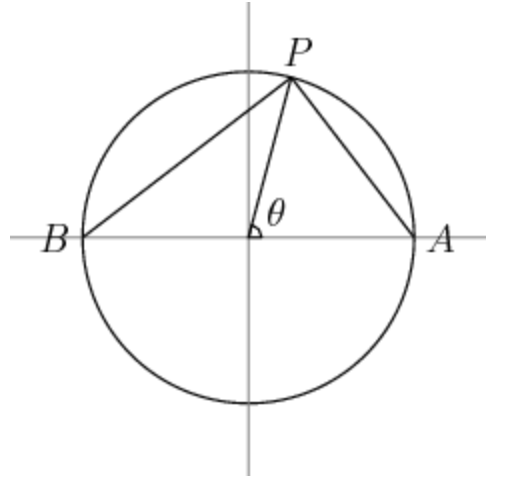# Expected Distance on a CircleAn angle $\theta$ is chosen randomly in the interval $[0,2\pi )$, and its corresponding point on the unit circle is plotted. Let this point be $P$. Now let $A=(1,0)$ and $B=(-1,0)$.

Let the expected value of $PA+PB-2$ be $E$.

What is $\lfloor 1000E\rfloor$?

×